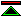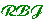# Is Hume's Principle Analytic?

### by George Boolos

This is a response to the final paragraph of the paper which I posted to the fom mailing list on 22nd April 2001.

In his paper entitled "Is Hume's Principle Analytic?" (reprinted in Logic, Logic and Logic) George Boolos concludes:

"It is thus difficult to see how on any sense of the word 'analytic', the key axiom of a theory that we don't know to be consistent and that contradicts our best established theory of number (on the natural readings of its primitives) can be thought of as analytic."
In this note I propose to show, contra-Boolos, how easy this can be.

The "key axiom" referred to is "HP" or "the cardinality principle". It is indeed difficult to see how this can be analytic if it does contradict the standard theory of cardinals, for it would then be in all probability false. I therefore propose to disregard Boolos's prejudicial referring phrase, and to show that HP understood as involving some concept of number distinct from the now standard notion of cardinal number, can easily be "thought of as analytic".

Though Boolos talks about "any sense" of the word analytic, it is clear from his paper that one sense he particularly has in mind is that in which analytic truths are described as "true in virtue of their meaning". I therefore proceed first by making this notion sufficiently precise that it is clearly well defined for certain classes of language which include at least one in which HP is analytic.

Clearly this sense of analyticity is only applicable to languages in which all the relevant parts are given a meaning, and is relative to a language and its semantics. It is possible that a sentence expressing HP (say "forall X,Y. #X=#Y <=> X =~ Y") may belong to more than one language, may have a meaning which varies from one to another, and may be analytic in some and false in others.

I therefore begin by defining the notion of "a semantics" for a first order language.

A semantics for a first order language is a non-empty set of interpretations of that language, which we will call "the intended" interpretations, and which are exactly those interpretations of the non-logical constants which are consistent with the (informally intended) semantics. A first order language together with a semantics for that language may be called an interpreted first order language.

We may now define analyticity for an interpreted first order language. A sentence in an interpreted first order language is analytic iff it is true in all the intended intepretations.

For the sake of discussing HP the above definition of analyticity can be extended to higher order logics with their standard semantics by replacing "first" by "higher-order" throughout.

I claim at this point that the concept of analyticity is as well defined as any concept in logic, and a great deal better defined than most in philosophy, and that this definition is, for the classes of languages in question, a very good (and the obvious) precise rendering of the informal notion "true in virtue of its meaning".

Though the concept of analyticity is now precisely and completely defined, its application depends upon the semantics of relevant languages being defined. In this I have in effect given a criterion for when the semantics is defined (i.e. when a certain non-empty set has been defined) without saying anything about how this should be done. It will be the case that the semantics of some languages (e.g. that of first order set theory) is not so easy to precisely define (or at least, to agree on how it should be defined). The concept of analyticity relative to a particular language will inherit any imprecision found in the semantics of the language.

A precise way of defining the semantics of a first or higher-order language is that advocated by David Hilbert. It is by giving a satisfiable set of ("non-logical") axioms which characterise the non-logical constants. The semantics is then the set of models of the axioms, and it follows immediately that all the theorems derivable in the logic from the axioms are analytic. In first order languages theoremhood and analyticity will then coincide, which tidy outcome is bought at a very heavy price, for most interesting mathematical concepts cannot be given quite the right semantics by a recursive axiomatisation in a first order language. (though an incomplete axiomatisation, i.e. one in which the set of "intended interpretations" contains some which are not really intended still yields a theory in which all theorems are analytic in the semantics you really wanted. The semantic gap corresponds to the proof theoretic one, there are some theorems which are true but not provable and it is these whose analyticity is misrepresented by an incomplete axiomatic semantics).

Alternative ways of defining the semantics are informal, or the use of an axiomatic semantics with informal codicils.

A classic example of a precise but informal semantics is that of the first order language of "true arithmetic". The semantics of this language may be specified by saying that it has just one intended interpretation (up to isomorphism) which is the natural numbers. The sentences of true arithmetic, under this semantics and the definition of analyticity given above, are analytic iff true, and under this terminology the truth of the logicist doctrine that "arithmetic is analytic" is established without any consideration of axioms.

A more full blooded logicism, the doctrine that "mathematics is analytic" can be approached through set theory. The simplest but semantically unsatisfactory method is to give a semantics to the language of first order set theory using the axioms of ZFC. Set theory and all the mathematics derivable in it then becomes analytic. Unfortunately this semantics is just plain wrong (in terms of the meaning of the mathematical concepts defined using it). There are at least two ways of fixing the semantics, each of which involves the injection of a degree of informality into the definition of the semantics. The first is to do set theory in second order logic (the "informality" here comes in the account of the standard semantics of second order logic). A second is to add an informal supplement to the first order axioms. For example, one might define the intended interpretations of first order set theory as "the well-founded models of ZFC". This is about the weakest/loosest semantics which is not obviously wrong. Doubtless set theorists, if they were interested, could come up with better definitions.

Returning to HP, it is now clear that under the proposed definitions we can define a language (call it "Frege arithmetic") which is a second order language with a "non-logical" function symbol "#", whose intended interpretations are exactly those in which HP is true under the standard second-order semantics. In this language, HP and all the truths of arithmetic which are derivable from it, are analytic (together with some which are not derivable). My own personal opinion is that this is a bad idea, HP providing a good basis neither for the semantics nor for the proof of arithmetic sentences. But it can be done.

Two subsidiary issues arise from the manner in which Boolos refers to HP in the quoted paragraph. The first is the mismatch between Frege's # and the usual notion of cardinality. This has no bearing on the analyticity of HP (under the above definitions) in any language in which it is true, but it does suggest that the recent tendency to prefer "the cardinality principle" as a name for HP may be misguided. The second is consistency. Under the above definitions there will be an interpreted second order language in which HP is analytic iff SOL+HP is consistent.

There are of course many other considerations raised in the paper which I have not here addressed, my aim here has been exclusively to comment on its final paragraph.

I invite philosophers of mathematics who subscribe to the alleged contemporary consensus against logicism to nominate the argument which they consider most decisively to refute that for logicism given in this note, and those philosophers who consider that HP provides a case for logicism which is otherwise absent to explain how and why the definition of analyticity provided here should be modified (or replaced) to make HP appear to have some relevance to the case for logicism.©created 2001-4-22 modified 2003-5-11